## Resource of the Week: Make 18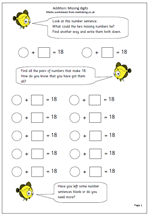Here is a worksheet that makes children think a little bit more. It shows a number sentence with two numbers that total 18. It asks what the two numbers could be.

The first thing to look for with this is children who use a logical or methodical approach. Usually children will write down the first answer that comes into their heads. That is why I have provided two blank number sentences at the top of the page. However, once they have done this they should start to revise their thinking and try to approach the task in an order so that they will know when they have reached all the possible answers.

This activity can be extended  using different numbers eg 19 or 20 and it is a useful exercise in helping children learn these pairs of numbers ‘off by heart’.

It can also be extended by allowing halves, which makes it quite a bit harder.

This can be found in the year 3 resources.

## Year 4 measurement word problems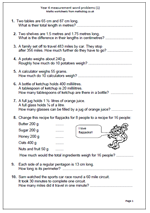Word problems can cause children quite a few problems as they have to work out what mathematical operation(s) to carry out to reach the right answers. This page is a set of word problems all to do with measurement, including time.

In order to reach the correct answers children need to be able to calculate and know about metric measurements. For example, question 1 asks:

Two tables are 65 cm and 87 cm long. What is their total length in metres.

Not only do they have to add 65 and 87 to get 152, but then convert this to 1.52 metres.

Other questions involve calculating in quarters and doubling the weight of the ingredients for making flapjacks; an early introduction to ratio.

Measuring word problems (1)

## Resource of the Week: written subtraction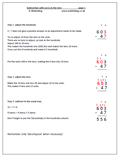This week I am looking at one of the hardest ideas to get across to children; carrying out a subtraction which requires adjusting across two columns. This only happens when there is a zero involved.

For example:

703

286 –

3 – 6 does not give a positive answer so an adjustment to the top number needs to be made. A ten can not be borrowed as there are none.

The procedure then is to borrow from the hundreds, move to the tens and then move again to the units. Often children will borrow from the hundreds, ignore the zero in the tens and move straight to the units. They cross out the 7 and make it 6 and make the 3 into 13.

This does not work as it has been 100 borrowed, not 10. Extra steps need to be taken by making the tens column 10 and then taking one ten, making this 9, and adjusting to the units.

Some children will see a quicker way: making the 70 one less (69) and adjusting to the units.

More explanation is available on the worksheet.

Standard subtraction with zeros in the tens (pg 1)

## Long multiplication: 3 by 2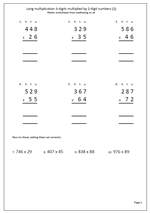I am often asked if there are more basic number sheets in the pipeline and so as to not disappoint people here is another page of long multiplication. The first six questions have been laid out in the correct way but the last four have been put in a horizontal position and will need laying out correctly.

The key with multiplying by 2-digit numbers is to multiply by the tens and then the units and finally add the two answers together. Some prefer to multiply by the units first: it makes no difference. Of course when multiplying by the tens digit it is important to remember to place a zero in the units column, ensuring that all other digits are then placed one place to the left and have a value ten times more.

It is also important to keep the numbers in straight columns to make adding up easier.

Long multiplication 3 by 2 (2)

## Resource of the week: division as sharing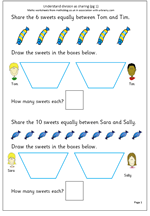Our resource of the week this week looks at early understanding of division. Division is usually the hardest of the four rules for children to learn, but in the early stages it is quite straightforward. Children often come across division for the first time when sharing, usually between two. The key concept,of course, is that the sharing is done equally. So, for example, 6 sweets shared between 2 children implies that the sharing is equal and they both receive the same number.

By far the best way to practice sharing is to use practical apparatus or use real life situations: e.g. share the strawberries equally between two, share or deal the cards equally etc. Usually this is done on a ‘one for you and one for me’ type process until there are none left. This maths worksheet replicates a practical situation, with the ultimate aim that children begin to remember the answers, which, of course are the inverse of multiplication.

Be careful when sharing that it is not always done into two, as some children begin to think that to share something it can always be divided by 2 and no other number.

Division as sharing (pg 1)

## Count back in threes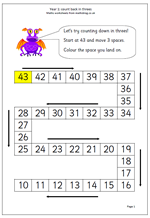Counting is one of those activities that it is all to easy to assume that children can do. In fact there are many children in Primary Schools who are very shaky in their knowledge of numbers and counting in ones, forwards or backwards. Yet one of the targets for Year 1 is for children to count on and back in twos and threes. This page is a help towards achieving this as it looks at counting back from a 2-digit number in threes. Starting at 43 count back 1, 2, 3 and reach 40; colour the square and count back a further three and so on. When the end of the line has been reached get children to read the numbers which have been coloured, both forwards and backwards. predictions could also be made as to which number comes next.

A blank sheet has been provided at the end of these pages so that different starting numbers can be used, or children asked to fill in the numbers themselves.

Count down in threes

## Maths Game: Pig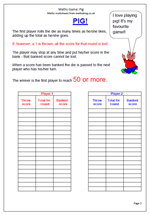This is a maths game more suited to upper primary school children as it requires quick mental arithmetic skills. The idea is to roll the die as many times as possible adding up the score as you go alon; but there is a catch!

Equipment:

A die

A scoring sheet is useful

Rules:

This is a game for two or more people, although usually played in pairs. It is good practice for addition skills up to 50, especially adding three or more small numbers.

The first player rolls the die as many times as he/she likes, adding up the total as he/she goes.

If, however, a 1 is thrown, all the score for that round is lost.

The player may stop at any time and put his/her score in the bank – that banked score cannot be lost.

When a score has been banked the die is passed to the next player who has his/her turn.

The winner is the first player to reach 50 or more.

Options: raise the winning score to 100 or more.

Use a 0-9 die with two losing options.

Pig

## Resource of the Week: Maths SAT questions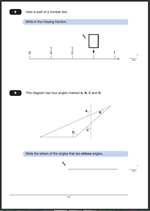For anyone in Year 6 the Maths SAT tests will be coming up before too long! Don’t forget that we have a whole section of past questions and answers, including great explanations of the best ways to approach each question. Invaluable help to achieve those higher levels!

This week I am highlighting are two questions from the Maths SAT Paper 2011 Paper B. The first involves equivalent fractions and the second angles and shape.

The answer to Question 8.   3/4
One mark awarded for a correct answer.
Equivalent fractions accepted as is 0.75.
Suggested method:
This question is another reading a number line problem and if children can count in quarters it is an easy mark. Firstly, it has to be recognised that the number line is showing quarters (1/2 is equivalent to 2/4 and 1 is equivalent to 4/4) making the missing number 3/4.

The answer to Question 9. A and D.
One mark awarded for a correct answer.
The letters can be given in either order. Both need to be given with no incorrect angles added.
Suggested method:
Answering this question correctly depends on 3 things:
Firstly, a recognition of the conventions for labelling angles, using the arc.
Secondly, a knowledge that an obtuse angle is larger than 90 degrees but less than 180 degrees.
If either of these are unknown it becomes purely a guessing game. (Some children do think that the angle depends on the length of the lines rather than a measure of turn.)

Thirdly, if these are known, then the obtuse angles still need to be recognised. One way to do this is to slide a right angle (eg a corner of a piece of paper) into the angle to see whether it is smaller or larger.

Why not visit ks2-maths-sats.co.uk for free SATs papers and a great SAT revision programme?

Questions 8 and 9 from SAT Paper B 2011

Questions 8 and 9 answers and suggested method 2011 Paper B

## Year 5 maths worksheet: solve single step word problems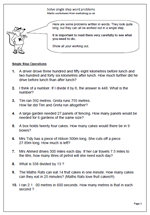This page contains a set of ten problems written in words. They look quite long, but they can all be worked out using a single step, using one of the operations: addition, subtraction, multiplication and division. Because they only require one step they are easier than questions which involve two or steps, but each calculation is quite tricky, with several needing a good knowledge of written methods to be able to do them.

It is important that all working out is shown for several reasons, including to show that a calculator was not used and to be able to spot where errors occur. Wrong answers are an excellent step towards new learning!

It is also interesting to see how children approach the questions. One or two questions in particular may cause problems. Question 6, involves subtracting of decimals and can be laid out in the standard format but could also be answered by adding on: e.g.

27.89 + 0.11 = 28

28 + 72 = 100

100 + 200 = 300

answer = 0.11 + 72 + 200 = 272.11

Solve single step word problems

## More adding three 2-digit numbers mentally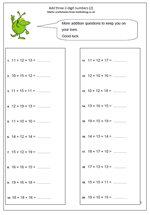I have had a number of requests for more on adding three 2-digit numbers mentally, so, here is another page. There are a number of different techniques which can be used when adding these and it certainly would not be the best way to proceed to just add them in the order they appear. Looking for pairs that make 30, doubling of teens, adjusting by 1 or 2 are just some of the skills needed to answer these quickly and accurately.

Also, don’t forget that correct answers are better than fast incorrect answers, so it is always a good idea to check by adding them in a different order.

Add 3 2-digit numbers practice (2)

This page can be found in the year 5 Calculating category, but many more addition pages can also be found in the four rules section.